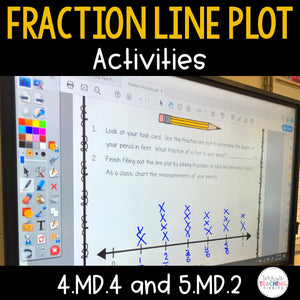# Fraction Line Plot Activities

• \$4.50
Unit price per
Shipping calculated at checkout.

Plotting fractions on a number line has never looked so fun! This pack comes with 3 whole group or small group activities for fraction line plots. Students are given individual task cards of an object measured on a fraction number line. As a class, each student will determine the fraction of their object and plot it onto a class line plot. Students will then use their class-created data and answer to answer questions.

Answer key is not included due to varying class sizes which would change the line plot data for each class.

This meets the 4th and 5th grade Common Core Standards and Georgia Standards of Excellence for fraction line plots.

Standards Included
4.MD.4 Make a line plot to display a data set of measurements in fractions of a unit (1/2, 1/4, 1/8). Solve problems involving addition and subtraction of fractions by using information presented in line plots.
5.MD.2 Make a line plot to display a data set of measurements in fractions of a unit (1/2, 1/4, 1/8). Use operations on fractions for this grade to solve problems involving information presented in line plots.

For more on fraction line plots, check out my Fraction Line Plot Packet HERE

## What You Get

• 3 sets of task cards (pencils, nails, and erasers)
• 28 task cards per set
• student record sheet with questions for each set of objects